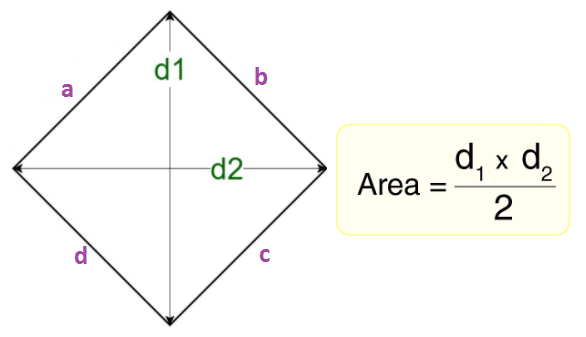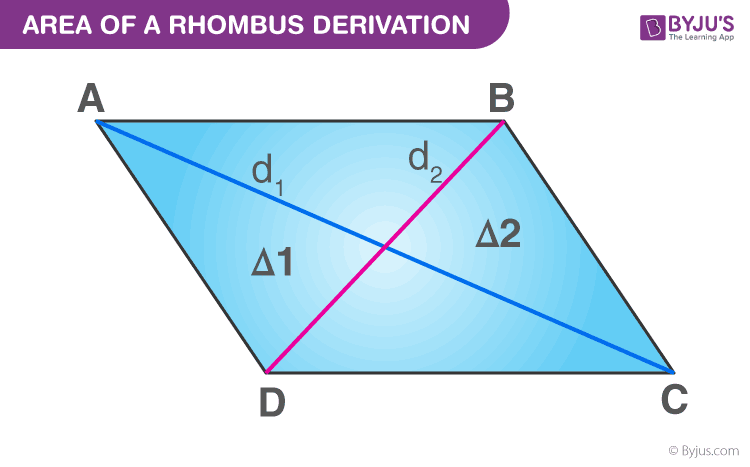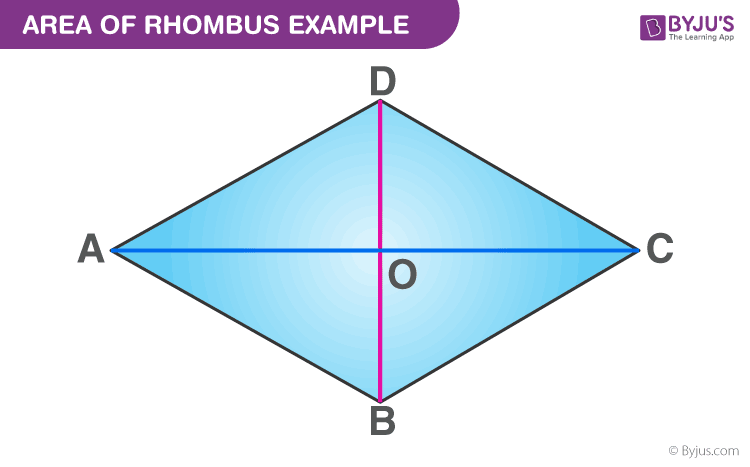Defend yourself better by mastering the science of immunity and vaccines. Secure your free spot, now! Defend yourself better by mastering the science of immunity and vaccines. Secure your free spot, now!

# Area Of Rhombus

In geometry, a rhombus is a special type of parallelogram in which two pairs of opposite sides are congruent. That means all the sides of a rhombus are equal. Students often get confused with square and rhombus. The main difference between a square and a rhombus is that all the internal angles of a square are right angles, whereas they are not right angles for a rhombus. In this article, you will learn how to find the area of a rhombus using various parameters such as diagonals, side & height, and side and internal angle, along with solved examples in each case.

## What is the Area of a Rhombus?

The area of a rhombus can be defined as the amount of space enclosed by a rhombus in a two-dimensional space. To recall, a rhombus is a type of quadrilateral projected on a two dimensional (2D) plane, having four sides that are equal in length and are congruent.

## Area of Rhombus Formula

Different formulas to find the area of a rhombus are tabulated below:

Formulas to Calculate Area of Rhombus
Using Diagonals A = ½ × d1 × d2
Using Base and Height A = b × h
Using Trigonometry A = b2 × Sin(a)

Where,

• d1 = length of diagonal 1
• d2 = length of diagonal 2
• b = length of any side
• h = height of rhombus
• a = measure of any interior angle## Derivation for Rhombus Area Formula

Consider the following rhombus: ABCDLet O be the point of intersection of two diagonals AC and BD.

The area of the rhombus will be:

A = 4 × area of ∆ AOB

= 4 × (½) × AO × OB sq. units

= 4 × (½) × (½) d1 × (½) d2 sq. units

= 4 × (1/8) d1 × d2 square units

= ½ × d1 × d2

Therefore, the Area of a Rhombus = A = ½ × d1 × d2

Where d1 and d2 are the diagonals of the rhombus.

Try This: Area of Rhombus Calculator

## How to Calculate Area of Rhombus?

The methods to calculate the area of a rhombus are explained below with examples. There exist three methods for calculating the area of a rhombus, they are:

• Method 1: Using Diagonals
• Method 2: Using Base and Height
• Method 3: Using Trigonometry (i.e., using side and angle)

### Area of Rhombus Using Diagonals: Method 1

Consider a rhombus ABCD, having two diagonals, i.e. AC & BD.

Step 1: Find the length of diagonal 1, i.e. d1. It is the distance between A and C. The diagonals of a rhombus are perpendicular to each other by making 4 right triangles when they intersect each other at the centre of the rhombus.

Step 2: Find the length of diagonal 2, i.e. d2 which is the distance between B and D.

Step 3: Multiply both the diagonals, d1, and d2.

Step 4: Divide the result by 2.

The resultant will give the area of a rhombus ABCD.

Let us understand more through an example.

Example 1: Calculate the area of a rhombus having diagonals equal to 6 cm and 8 cm.

Solution:

Given that,

Diagonal 1, d1 = 6 cm

Diagonal 2, d2 = 8 cm

Area of a rhombus, A = (d1 × d2) / 2

= (6 × 8) / 2

= 48 / 2

= 24 cm2

Hence, the area of the rhombus is 24 cm2.

### Area of Rhombus Using Base and Height: Method 2

Step 1: Find the base and the height of the rhombus. The base of the rhombus is one of its sides, and the height is the altitude which is the perpendicular distance from the chosen base to the opposite side.

Step 2: Multiply the base and the calculated height.

Let us understand this through an example:

Example 2: Calculate the area of a rhombus if its base is 10 cm and height is 7 cm.

Solution:

Given,

Base, b = 10 cm

Height, h = 7 cm

Area, A = b × h

= 10 × 7 cm2

A = 70 cm2

### Area of Rhombus Using Trigonometry: Method 3

This method is used to calculate the area of the rhombus when the side and one of its internal angles are given.

• Step 1: Square the length of any of the sides.
• Step 2: Multiply it by Sine of one of the angles.

Let us see how to find the area of a rhombus using the side and angle in the below example.

Example 3: Calculate the area of a rhombus if the length of its side is 2 cm and one of its angles A is 30 degrees.

Solution:

Given,

Side = s = 2 cm

Angle A = 30 degrees

Square of side = 2 × 2 = 4

Area, A = s2 × sin (30°)

A = 4 × 1/2

A = 2 cm2

### Solved Problem on Area of Rhombus Formula

Question: Find the area of the rhombus having each side equal to 17 cm and one of its diagonals equal to 16 cm.

Solution:Area of Rhombus Example Question

ABCD is a rhombus in which AB = BC = CD = DA = 17 cm

Diagonal BD = 16 cm (with O being the diagonal intersection point)

Therefore, BO = OD = 8 cm

In ∆ AOD,

⇒ 172 = AO2 + 82

⇒ 289 = AO2 + 64

⇒ 225 = AO2

⇒ AO = 15 cm

Therefore, AC = 2 × AO

= 2 × 15

= 30 cm

Now, the area of the rhombus

= ½ × d1 × d2

= ½ × 16 × 30

= 240 cm2

### Practice Questions

1. Find the height of the rhombus, whose area is 175 cm² and perimeter is 100 cm.
2. Calculate the area of a rhombus with a side of 5 cm, and one of the internal angles is 120 degrees.
3. If the area of a rhombus is 143 sq. units and one of its diagonal is 26 units, find the other diagonal.

Q1

### What is a Rhombus?

A rhombus is a type of quadrilateral whose opposite sides are parallel and equal. Also, the opposite angles of a rhombus are equal and the diagonals bisect each other at right angles.

Q2

### What is the Formula for Area of a Rhombus?

To calculate the area of a rhombus, the following formula is used:

A = ½ × d1 × d2

Q3

### How to Find the Area of a Rhombus When the Side and Height are Given?

To find the area of a rhombus when the measures of its height and side are given, use the following formula:

A = Base × Height

Q4

### What is the Formula for Perimeter of a Rhombus?

The formula to calculate the perimeter of a rhombus of side “a” is:

P = 4a units

Q5

### How to find the area of a rhombus if one of its sides and an included angle are given?

If “a” be its sides and “θ” is an included angle, then the formula is:
Area of a Rhombus = a2 sin θ square units.

Q6

### Find the area of a rhombus if the diagonal measures 6cm and 4cm.

We know that, Area of Rhombus = (½) × Diagonal 1 × Diagonal 2
Substituting the values, we get
A = (½) × 4 × 6 = 12 cm2.

Q7

### Is the area of a rhombus the same as the area of a square?

No, the area of a rhombus is not the same as the area of a square.

Q8

### What is the difference between the area of a rhombus and the area of a square?

The area of a square is the square of its side, whereas the area of a rhombus is the half the product of diagonal 1 and diagonal 2.